Essential Concept 76: FRA Pricing and Valuation | IFT World
101 Concepts for the Level I Exam

# Essential Concept 76: FRA Pricing and ValuationA forward rate agreement is an over-the-counter forward contract in which the underlying is an interest rate. FRAs are usually expressed in the “X x Y” convention, ‘X’ represents the point where the underlying loan starts. This also the point where the FRA expires. ‘Y’ represents the point where the underlying loan ends. A 3 x 9 FRA is depicted in the figure below.

The FRA fixed rate i.e. price of an FRA can be calculated using these steps:

1. Set up the time line.
2. Compute the de-annualized fixed rate: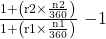3. Annualize by multiplying by 360/(n2 – n1)

The FRA value for pay fixed receive floating can be calculated using these steps:

1. Find the new FRA fixed rate at time t
2. Compute payoff at n2: (FRAt – FRA0) x (n2 – n1)/360 x NP
3. Discount back to time t.

Example: Suppose we entered a receive-floating 6×9 FRA at a rate of 0.89%, with a notional amount of \$5,000,000 at T = 0. After 90 days, the three-month US dollar Libor is 1.10% and the six-month US dollar Libor is 1.25% which will be the discount rate to determine the value. What is the value of the original receive-floating 6×9 FRA?

Solution:

1. We first compute the new FRA rate at time t.

Step 1: Set up the time line.

Step 2: Compute the de-annualized fixed rate: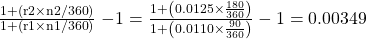Step 3: Annualize by multiplying by 360/(n2 – n1)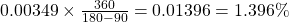1. Compute payoff at n2: (FRAt – FRA0) x (n2 – n1)/360 x NP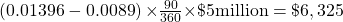2. Discount back to time t.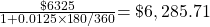Zoom Classes for Nov-23 Exam Starting Now!
This is default text for notification bar